# Cuboid box

How many m2 paper is needed for the sticking cuboid box of dimensions 50 cm, 40 cm and 30 cm? To the folds add one-tenth the area.

x =  1.034 m2

### Step-by-step explanation: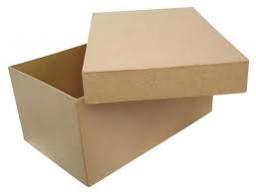Did you find an error or inaccuracy? Feel free to write us. Thank you!Tips to related online calculators
Need help to calculate sum, simplify or multiply fractions? Try our fraction calculator.
Do you want to convert area units?
Do you want to convert length units?

#### You need to know the following knowledge to solve this word math problem:

We encourage you to watch this tutorial video on this math problem:

## Related math problems and questions:

• Metal sheet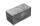How much m ^ 2 of sheet metal is needed to beat a box in the shape of a cube with an edge length of 50 cm, if we add 20% on the folds of the lid and walls?
• Metal box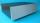How much metal we need for the production of box with dimensions 5 dm, 30 cm and a height of 1 m? Add 12% of the waste and folds.
• Glass aquarium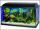How many m2 of glass are needed to produce an aquarium with bottom dimensions of 70 cm x 40 cm and 50 cm high?
• Paper boxCalculate whether 11 dm² of paper is sufficient for gluing a box without a lid with bottom dimensions of 2 dm and 15 cm and 12 cm high. Write result as: 0 = No, 1 = Yes
• PresentGift box has a rectangular shape with dimensions of 8×8×3 cm. Miloslav wants to cover with square paper with sides of 18 cm. How much paper left him?
• Cuboid box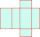Cuboid box have dimensions of 30 cm, 25 cm and 40 cm. Sketch its network and compute surface area of box.
• Concrete boxThe concrete box with walls thick 7 cm has the following external dimensions: length 1.8 m, width 44 cm and height 46 cm. How many liters of soil can fit if I fill it to the brim?
• Paper box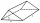Calculate how much we'll pay for a three-side shaped prism box with a triangular base, and if it measures 12cm and 1.6dm, the hypotenuse measures 200mm. The box is 34cm high. We pay 0,13 € per square meter of paper.
• CardboardHow many m2 of cardboard are needed to make the cuboid with dimensions 40 cm 60 cm and 20 cm?
• AquariumThe box-shaped aquarium is 40 cm high; the bottom has dimensions of 70 cm and 50 cm. Simon wanted to create an exciting environment for the fish, so he fixed three pillars to the bottom. They all have the shape of a cuboid with a square base. The base edg
• Insulate houseThe property owner wants to insulate his house. The house has these dimensions 12, and 12 m is 15 m high. The windows have 6 with dimensions 170 and 150 cm. Entrance doors are 250 and 170 cm in size. How many square meters of polystyrene does he need?
• TableclothsThe restaurant has sixty-two square tablecloths with a side length of 150 cm and 36 rectangular tablecloths with dimensions of 140 cm and 160 cm. A) How many meters of hemming ribbon will be needed if we add 50 cm to each tablecloth? B) The ribbon sale in
• Empty aquariumHow much does an empty aquarium weigh with dimensions: length = 40 cm, width = 30 cm, height = 20 cm, if 1 dm2 of glass weighs 300 g? Calculate its weight in kilograms.
• Milk cartonsHow much paper do we need for 12 tetra packs with dimensions 6 cm, 11 cm, and 20 cm? Will 1 liter of milk fit in the box?
• Surface and volumeFind the surface and volume of a cuboid whose dimensions are 1 m, 50 cm, and 6 dm.
• CapJesters hat is shaped by a rotating cone. Calculate how much paper is needed to the cap 54 cm high when the head circumference is 47 cm.
• Paper box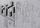Calculate the consumption of paper on the box-shaped quadrangular prism with rhombic footstall, base edge a=6 cm and the adjacent base edges forms an angle alpha = 60 °. Box height is 10 cm. How many m2 of the paper consumed 100 such boxes?